# Maharashtra Board 11th Maths Solutions Chapter 4 Determinants and Matrices Ex 4.7

Balbharti Maharashtra State Board Class 11 Maths Solutions Pdf Chapter 4 Determinants and Matrices Ex 4.7 Questions and Answers.

## Maharashtra State Board 11th Maths Solutions Chapter 4 Determinants and Matrices Ex 4.7

Question 1.
Find AT, if
i. A = $$\left[\begin{array}{cc} 1 & 3 \\ -4 & 5 \end{array}\right]$$
ii. A = $$\left[\begin{array}{ccc} 2 & -6 & 1 \\ -4 & 0 & 5 \end{array}\right]$$
Solution:
i. A = $$\left[\begin{array}{cc} 1 & 3 \\ -4 & 5 \end{array}\right]$$
∴ AT = $$\left[\begin{array}{rr} 1 & -4 \\ 3 & 5 \end{array}\right]$$ii. A = $$\left[\begin{array}{ccc} -4 & 0 & 5 2 & -6 & 1 \\ \end{array}\right]$$
∴ AT = $$\left[\begin{array}{cc} 2 & -4 \\ -6 & 0 \\ 1 & 5 \end{array}\right]$$

[Note: Answer given in the textbook is AT = $$\left[\begin{array}{cc} 2 & -4 \\ 6 & 0 \\ 1 & 5 \end{array}\right]$$. However, as per our calculation it is AT = $$\left[\begin{array}{cc} 2 & -4 \\ -6 & 0 \\ 1 & 5 \end{array}\right]$$. ]

Question 2.
If [aij]3×3 where aij = 2(i – j), find A and
AT. State whether A and AT are symmetric or skew-symmetric matrices?
Solution:
A = [aij]3×3 = $$\left[\begin{array}{lll} a_{11} & a_{12} & a_{13} \\ a_{21} & a_{22} & a_{23} \\ a_{31} & a_{32} & a_{33} \end{array}\right]$$
Given aij = 2 (i — j)
∴ a11 = 2(1-1) = 0,
a12 = 2(1-2) = -2,
a13 = 2(1-3) = -4,
a21 = 2(2-1) = 2,
a22 = 2(2-2) = 0,
a23=2(2-3) = -2,
a31 = 2(3-1) = 4,
a32 = 2(3-2) = 2,
a33=2(3-3) = 0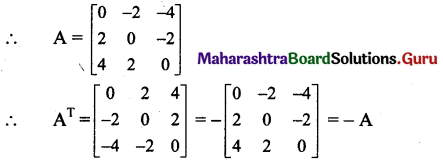∴ AT = -A and A = -AT
∴ A and AT both are skew-symmetric matrices.

Questionn 3.
If A = $$\left[\begin{array}{cc} 5 & -3 \\ 4 & -3 \\ -2 & 1 \end{array}\right]$$, prove that (2A)T = 2AT.
Solution: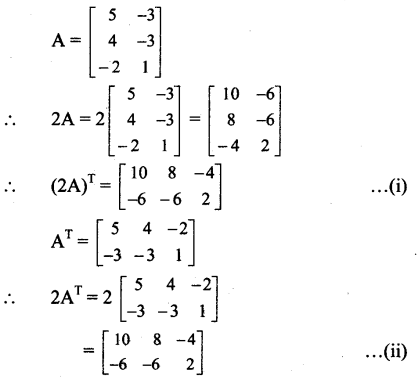From (i) and (ii), we get
(2A)T = 2ATQuestion 4.
If A = $$\left[\begin{array}{ccc} 1 & 2 & -5 \\ 2 & -3 & 4 \\ -5 & 4 & 9 \end{array}\right]$$, prove that (3A)T = 3AT.
Solution: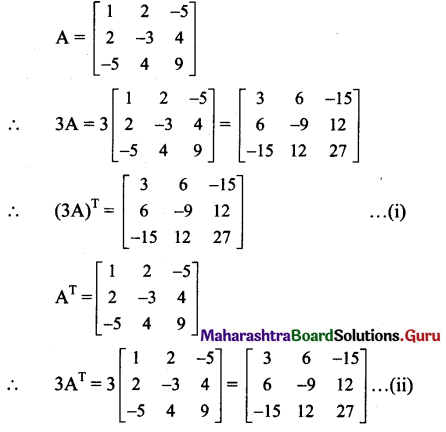From (i) and (ii), we get
(3A)T = 3AT

Question 5.
If A = $$\left[\begin{array}{ccc} 0 & 1+2 i & 1-2 \\ -1-2 i & 0 & -7 \\ 2-i & 7 & 0 \end{array}\right]$$,
where i = $$\sqrt{-1}$$, prove that AT = – A.
Solution: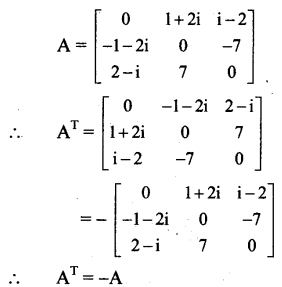Question 6.
If A = $$\left[\begin{array}{cc} 2 & -3 \\ 5 & -4 \\ -6 & 1 \end{array}\right]$$ , B = $$\left[\begin{array}{cc} 2 & 1 \\ 4 & -1 \\ -3 & 3 \end{array}\right]$$ and C = $$\left[\begin{array}{cc} 1 & 2 \\ -1 & 4 \\ -2 & 3 \end{array}\right]$$ then show that
i. (A + B)T = AT + BT
ii. (A – C)T = AT – CT
Solution: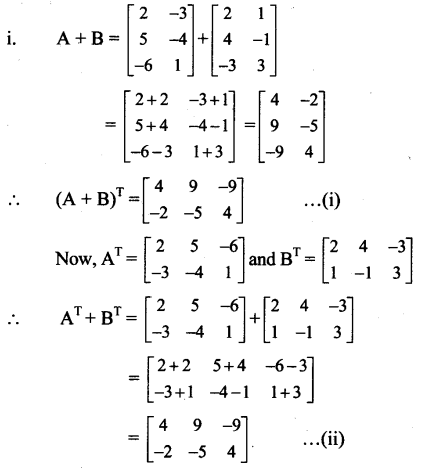From (i) and (ii), we get
(A + B)T = AT + BT
[Note: The question has been modified.]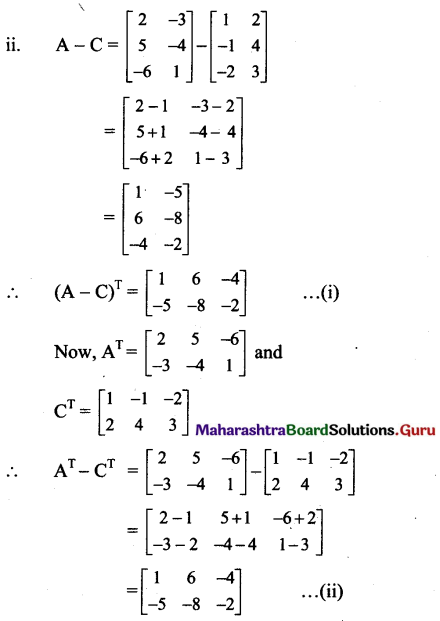From (i) and (ii), we get
(A – C)T = AT – CT</supQuestion 7.
If A = $$\left[\begin{array}{cc} 5 & 4 \\ -2 & 3 \end{array}\right]$$ and $$\left[\begin{array}{cc} -1 & 3 \\ 4 & -1 \end{array}\right]$$ then find CT, such that 3A – 2B + C = I, where I is the unit matrix of order 2.
Solution:
3A – 2B + C = I
∴ C = I + 2B – 3A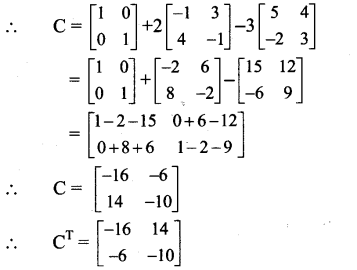Question 8.
If A = $$\left[\begin{array}{ccc} 7 & 3 & 0 \\ 0 & 4 & -2 \end{array}\right]$$, B = $$\left[\begin{array}{ccc} 0 & -2 & 3 \\ 2 & 1 & -4 \end{array}\right]$$, then find
i. AT + 4BT
ii. 5AT – 5BT
Solution: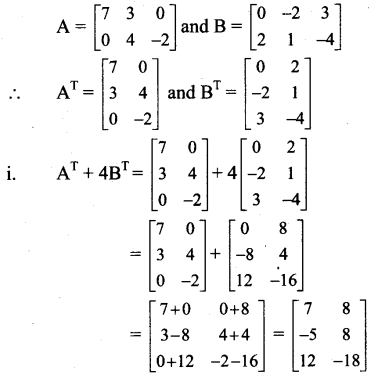ii. ii. 5AT – 5BT = 5(AT – BT)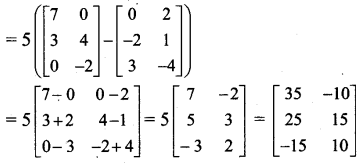Question 9.
If A = $$\left[\begin{array}{lll} 1 & 0 & 1 \\ 3 & 1 & 2 \end{array}\right]$$, B = $$\left[\begin{array}{rrr} 2 & 1 & -4 \\ 3 & 5 & -2 \end{array}\right]$$ and C = $$\left[\begin{array}{ccc} 0 & 2 & 3 \\ -1 & -1 & 0 \end{array}\right]$$, verify that (A + 2B + 3C)T = AT + 2BT + 3CT.
Solution:
A + 2B + 3C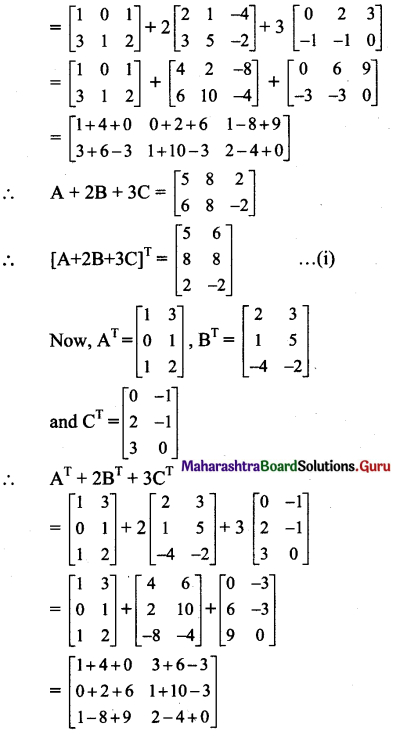∴ AT + 2BT + 3CT = $$\left[\begin{array}{cc} 5 & 6 \\ 8 & 8 \\ 2 & -2 \end{array}\right]$$
From (i) and (ii), we get
(A + 2B + 3C)T = AT + 2BT + 3CTQuestion 10.
If A = $$\left[\begin{array}{ccc} -1 & 2 & 1 \\ -3 & 2 & -3 \end{array}\right]$$ and B = $$\left[\begin{array}{cc} 2 & 1 \\ -3 & 2 \\ -1 & 3 \end{array}\right]$$, prove that (A + BT)T = AT + B.
prove that (A + BT)T = AT + B
Solution: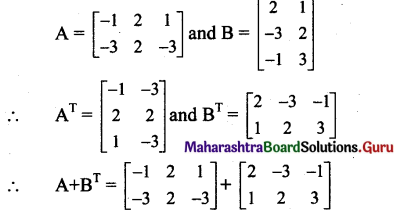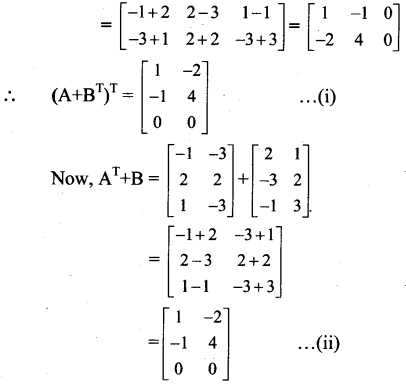From (i) and (ii), we get
(A + BT)T = AT + B

Question 11.
Prove that A + AT is a symmetric and A – AT is a skew symmetric matrix, where
i. A = $$\left[\begin{array}{ccc} 1 & 2 & 4 \\ 3 & 2 & 1 \\ -2 & -3 & 2 \end{array}\right]$$
ii. A = $$\left[\begin{array}{ccc} 5 & 2 & -4 \\ 3 & -7 & 2 \\ 4 & -5 & -3 \end{array}\right]$$
Solution: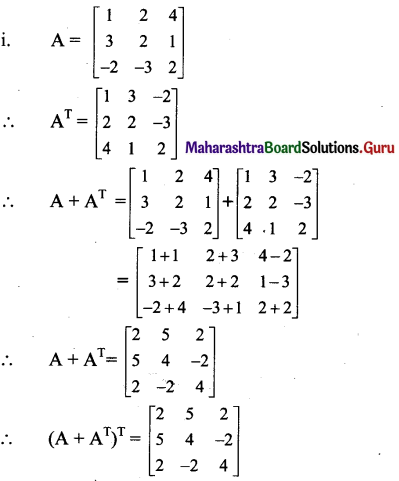∴ (A + AT)T = A + AT, i.e., A + AT = (A + AT)T
∴ A + AT is a symmetric matrix.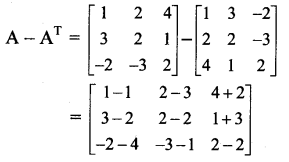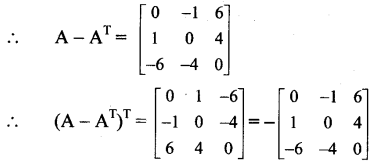∴ (A – AT)T = – (A – AT),
i.e., A – AT = -(A – AT)T
∴ A – AT is skew symmetric matrix.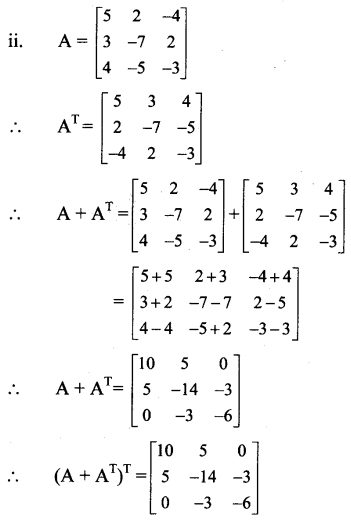∴ (A + AT)T = A + AT, i.e., A + AT = (A + AT)T
∴ A + AT is a symmetric matrix.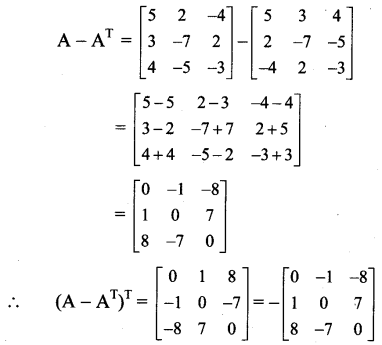∴ (A – AT)T = – (A – AT),
i.e., A – AT = -(A – AT)T
∴ A – AT is skew symmetric matrix.Question 12.
Express the following matrices as the sum of a symmetric and a skew symmetric matrix.
i. $$\left[\begin{array}{cc} 4 & -2 \\ 3 & -5 \end{array}\right]$$
ii. $$\left[\begin{array}{ccc} 3 & 3 & -1 \\ -2 & -2 & 1 \\ -4 & -5 & 2 \end{array}\right]$$
Solution:
A square matrix A can be expressed as the sum of a symmetric and a skew symmetric matrix as
A = $$\frac{1}{2}$$ (A + AT) + $$\frac{1}{2}$$ (A – AT)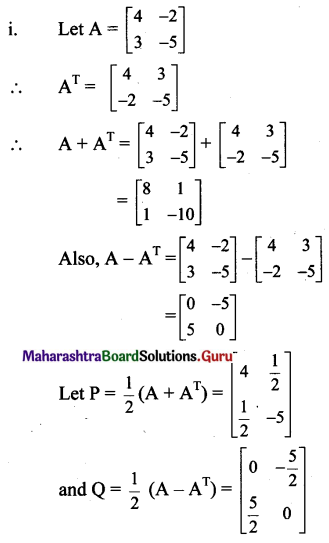P is symmetric matrix …[∵ aij = aji]
and Q is a skew symmetric matrix [∵ -aij = -aji]
A = P + Q
A = $$\left[\begin{array}{cc} 4 & \frac{1}{2} \\ \frac{1}{2} & -5 \end{array}\right]+\left[\begin{array}{ll} 0 & \frac{-5}{2} \\ \frac{5}{2} & 0 \end{array}\right]$$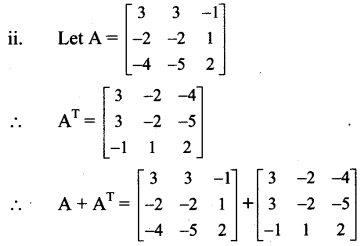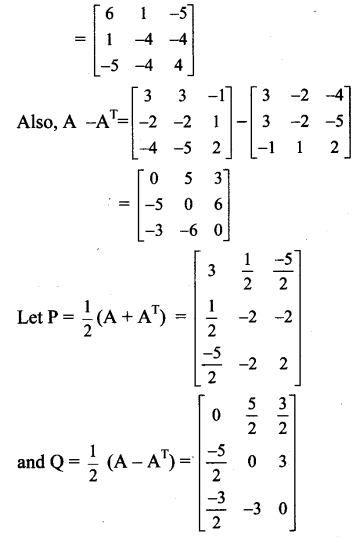∴ P is symmetric matrix …[∵ aij = aji]
and Q is a skew symmetric matrix [∵ -aij = -aji]
∴ A = P + Q
∴ A = $$\left[\begin{array}{cc} 4 & \frac{1}{2} \\ \frac{1}{2} & -5 \end{array}\right]+\left[\begin{array}{ll} 0 & \frac{-5}{2} \\ \frac{5}{2} & 0 \end{array}\right]$$

Question 13.
If A = $$\left[\begin{array}{cc} 2 & -1 \\ 3 & -2 \\ 4 & 1 \end{array}\right]$$ and B = $$\left[\begin{array}{ccc} 0 & 3 & -4 \\ 2 & -1 & 1 \end{array}\right]$$, verify that
i. (AB)T = BTAT
ii. (BA)T = ATBT
Solution: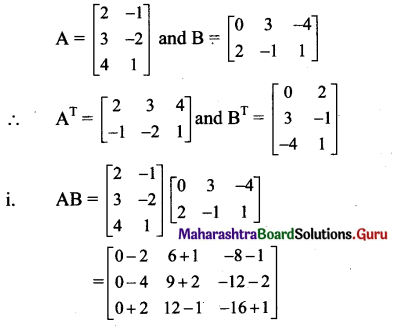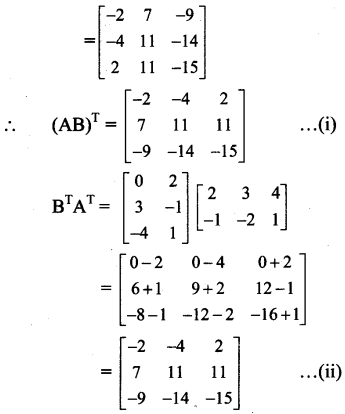From (i) and (ii), we get
(AB)T = BTAT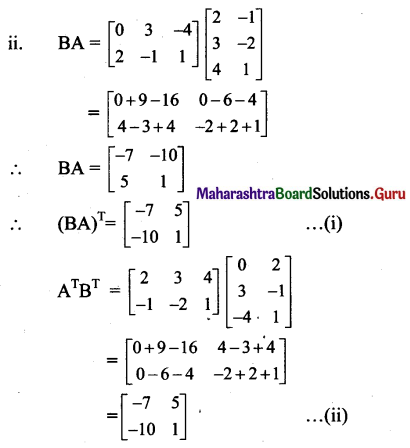From (i) and (ii) we get
(BA)T = ATBTQuestion 14.
If A = $$\left[\begin{array}{cc} \cos \alpha & \sin \alpha \\ -\sin \alpha & \cos \alpha \end{array}\right]$$, show that ATA = I, where I is the unit matrix of order 2.
Solution: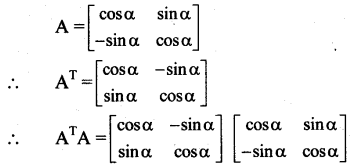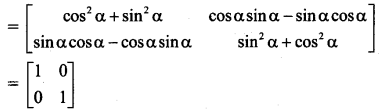∴ ATA = I, where I is the unit matrix of order 2.